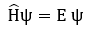Classical mechanics: is based on Newton’s laws of motion. It successfully describes the motion of macroscopic particles but fails in the case of microscopic particles.

Reason: Classical mechanics ignores the concepts of the dual behaviour of matter especially for sub-atomic particles and the Heisenberg’s uncertainty principle.

Quantum mechanics: is a theoretical science that deals with the study of the motions of the microscopic objects that have both observable wavelike and particle-like properties.

Quantum mechanics is based on a fundamental equation which is called the Schrodinger equation.H is a mathematical operator called Hamiltonian.

E is the total energy of the system.

Ψ represents the wave function which is the amplitude of the electron wave.

# Schrodinger’s equation

For a system (such as an atom or a molecule whose energy does not change with time) the Schrodinger equation is written as:

When Schrodinger equation is solved for the hydrogen atom, the solution gives the possible energy levels the electron can occupy and the corresponding wave function(s) of the electron associated with each energy level. Out of the possible values, only certain solutions are permitted. Each permitted solution is highly significant as it corresponds to a definite energy state. Thus, we can say that energy is quantized.

Probability density: Ψ gives us the amplitude of the wave. The value of Ψ has no physical significance. Ψ2 gives us the region in which the probability of finding an electron is maximum. It is called probability density.

Orbital: The region of space around the nucleus where the probability of finding an electron is maximum is called an orbital.